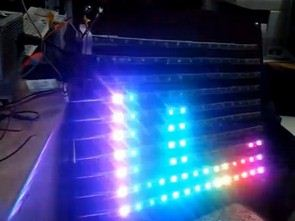# Arduino 50V digital Voltmeter using MAX7219

### Abstract

The Arduino inbuilt 10 bit ADC, can be used for constructing / measuring the 0Volt to 50Volt Digital Volt Meter. Popular MAX7219 display driver, connected with Arduino Uno will be used for displaying the measured voltage. This voltmeter can read only DC voltage.### Parts and components

• Arduino Uno board
• Max7219 4 Digit 7Segment Common cathode displays = 1 Nos
• Or Max7219 assembled board
• Resistor 27 K = 1 No
• Resistor 1.2M = 1 No
• IN5913B 3.3 Volt Zener diode = 1 No

## Step 1: Schematic

• The MAX7219 display driver chip provides a 3-wire serial (SPI) interface to drive 7-segment LED displays (common-cathode type) up to 8 digits.
• The on-chip includes a BCD decoder, multiplex scan circuitry, segment and digit drivers, and an 8×8 static RAM to store the digit values.
• The DIN, LOAD and CLOCK pins of MAX7219 is connected with 11,10 and 9 digital IO pins of Arduino.
• A four digit, common cathode seven segment display is connected to the drive pins of MAX7219.
• Otherwise, one can use the pre-assembled MAX7219 board with 4 digits, and connect the drive pins accordingly.
• Voltage divider circuit is used to divide voltage into two parts (measurement and drop).
• Internal Vref (1100 mV) of Arduino is selected for measurement.
• The resistor R3 (1200K) and R2 (27K) forms the voltage divider, and connected to analog input pin of Arduino (A0).
• IN5913B 3.3 Volt Zener diode, at A0 pin, will product the Arduino, from over voltage inputs.
• Vmeasurment = (R2 / R3+R2 ) * Vin.
• If Vin is 50V then Vmeasurment = (27/1227) * 50V = 1.1 V = 1100 mV.
• ADC Resolution = Vref / (1024 -1).
• ADC Resolution = 1.1 V / 1023 = 1100mV / 1023 = 1.075 mV.
• DVM Resolution = 50 / 1023 = 48.875 mV.

## Step 2: Software

• The Arduino LedControl library is used for displaying digits on MAX7219.
• The 1.1V internal reference is chosen for the ADC.
• The voltage divider will, provide the input voltage between 0 to 1100mV.
• Hence the software will measure and display the values between 00.00 Volt to 50.00 Volt.
• 10 bit ADC reading is mapped to 1100 mV, which is the Vref mille volt.

#### This Post / Project can also be found using search terms:

• arduino voltmètre à led